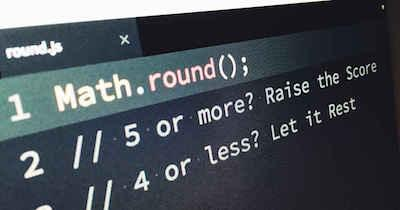# How To Round Numbers In Javascript

There’s no question that rounding numbers can be very useful. However, sometimes, you may want to use a different language to round your numbers.

While it is fairly easy to round numbers manually, you may be looking for the best way to round numbers in javascript. So, this is what we’re going to show how to do today.

## How To Round Numbers In Javascript

When you want to round numbers in javascript to its nearest integer, you need to use the Math.round() function.

Here’s the syntax of the function:

Math.round(value)

• Parameters: the number to be rounded to its nearest integer.
• Returns: Result after rounding the number passed as a parameter to the function passed as parameter.

Let’s see some examples so you can easily understand how to apply the function.

Learn how to round numbers in java.

## #1: Rounding Off A Number To Its Nearest Integer:

To round off a number to its nearest integer, the math.round() function should be implemented in the following way:

<script type=”text/javascript”>

var round =Math.round(5.8);

document.write(“Number after rounding : ” + round);

</script>

When you run the function, the output is 6.

Discover how to round numbers in python.

## #2: Rounding Off A Negative Number To Its Nearest Integer:

One of the best things about using this Math.round() function is that it rounds off a negative number when passed as a parameter to it. So, to round off a negative number to its nearest integer, the Math.round() function should be implemented in the following way:

<script type=”text/javascript”>

var round =Math.round(-5.8);

document.write(“Number after rounding : ” + round);

</script>

When you run the function, you will get an output of -6.

## #3: Math.round() Function, When Parameter Has “.5” As Decimal:

In this case, you need to use the following code:

<script type=”text/javascript”>

var round =Math.round(-12.5);

document.write(“Number after rounding : ” + round);

var round =Math.round(12.51);

document.write(“Number after rounding : ” + round);

</script>

In this case, you will get two different outputs. The first output is -12, and the second output is 13.

## How To Round Numbers In Javascript – Errors And Exceptions

The truth is that up until this point, you shouldn’t have any questions about the function we are using to round numbers. Nevertheless, you need to ensure that you know there are some errors and exceptions to this formula:

• A non-numeric string passed as a parameter returns NaN
• An array with more than 1 integer passed as a parameter returns NaN
• An empty variable passed as a parameter returns NaN
• An empty string passed as a parameter returns NaN
• An empty array passed as a parameter returns NaN.

Below are some examples that illustrate the Math.floor() function in JavaScript:

## #1: Code:

<!– NEGATIVE NUMBER EXAMPLE –>

<script type=”text/javascript”>

document.write(Math.round(-2));

document.write(Math.round(-2.56));

</script>

In this case, the outputs are going to be -2 and -3.

## #2: Code:

<!– POSITIVE NUMBER EXAMPLE –>

<script type=”text/javascript”>

document.write(Math.round(2));

document.write(Math.round(2.56));

</script>

In this case, the outputs are going to be 2 and 3.

## #3: Code:

<!– STRING EXAMPLE –>

<script type=”text/javascript”>

document.write(Math.floor(“Geeksforgeeks”));

</script>

In this case, the output is NaN.

## #4: Code:

<!– ADDITION INSIDE FUNCTION EXAMPLE –>

<script type=”text/javascript”>

document.write(Math.floor(7.2+9.3));

</script>

In this case, the output is 17.